Area of Trapezium

Chapter 9 Class 8 Mensuration
Concept wise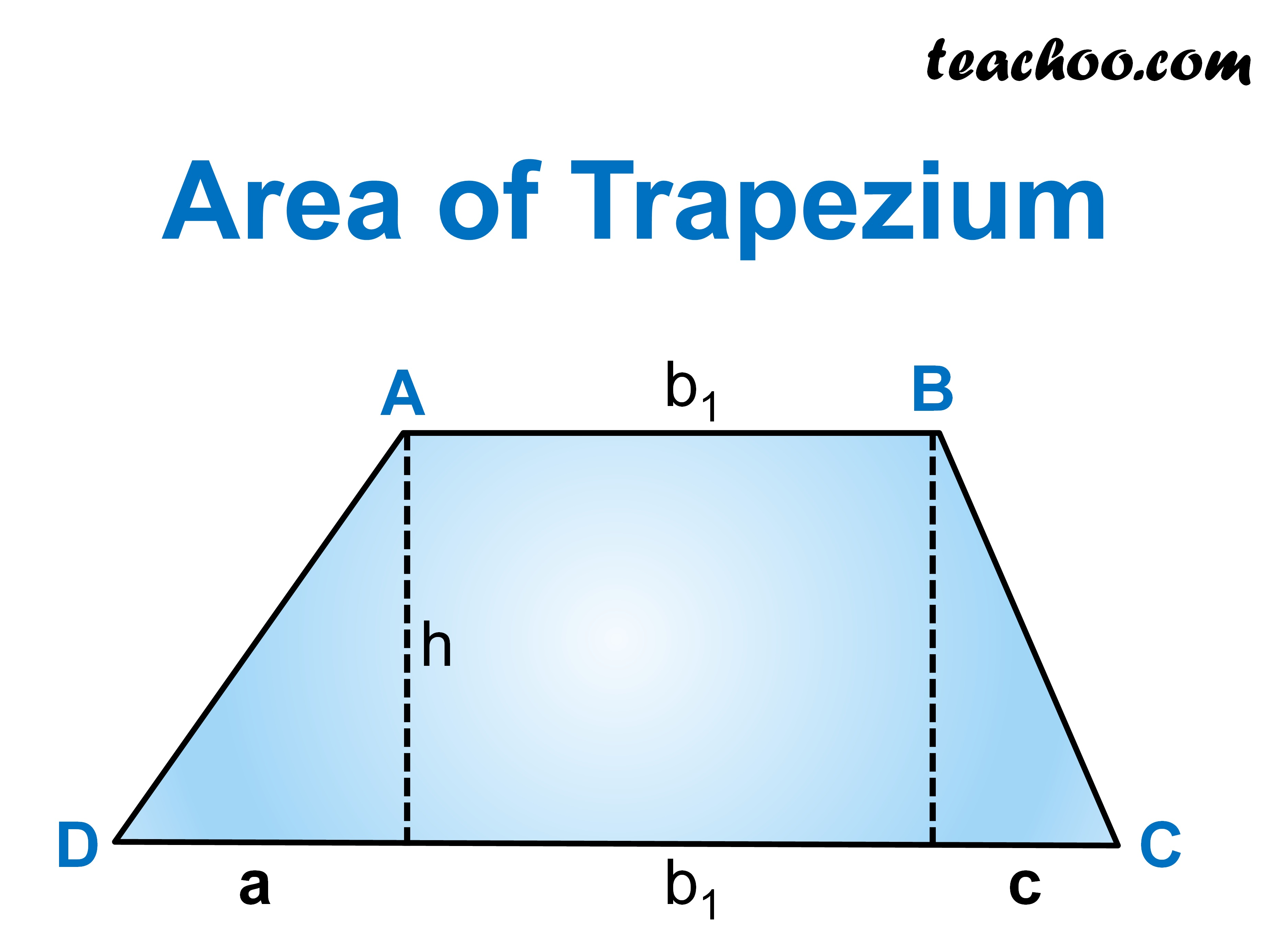A trapezium is a quadrilateral with one pair of opposite

sides parallel

So, in trapezium ABCD

AB ∥ CD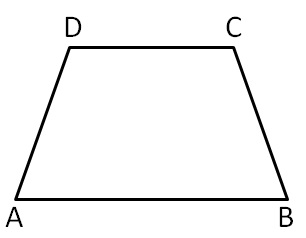Area of trapezium is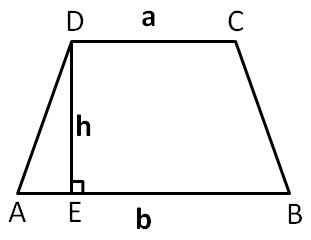Area of trapezium = 1/2 × Sum of parallel sides × Height

= 1/2 × (a + b) × h

Here,

Height is perpendicular distance between two parallel lines

#### Find area of trapezium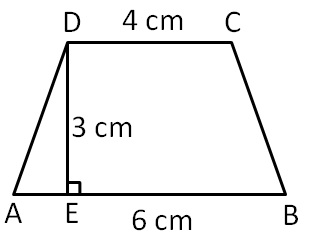Here,

Parallel sides are 4 cm and 6 cm

and height is 3 cm

So, a = 4 cm, b = 6 cm and h = 3 cm

Area of trapezium = 1/2 (a + b) × h

= 1/2 × (6 + 4) × 3

= 1/2 × 10 × 3

= 5 × 3 = 15 cm 2

#### Find height of trapezium if parallel sides are 4 cm and 6 cm and area of trapezium is 15 cm 2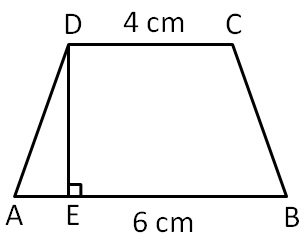Here,

parallel sides are 4 cm and 6 cm

So, a = 4 cm, b = 4 cm

Area of trapezium = 1/2 (a + b) × h

15 = 1/2 × (6 + 4) × h

15 = 1/2 × 10 × h

15 = 5h

15/5 = h

h = 3 cm

Learn in your speed, with individual attention - Teachoo Maths 1-on-1 Class